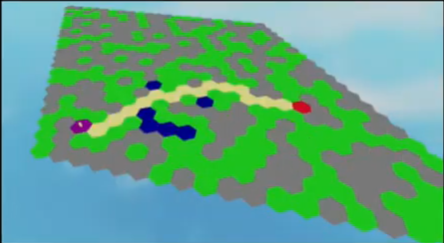# What's the most effective way to script a following pathfinding AI?

I’m pretty new to the pathfinding service, but I have a general grasp of how it works. I have a (somewhat) working AI that follows you which utilizes the service, walking around obstacles to get to the target. However, the movement is very buggy once you start to move.

I’m using for loops to sift through waypoints and have the NPC move to that point. When the target moves, the path is recomputed and the new waypoints are reset (and in this process, the waypoint loop breaks to prevent previous waypoints affecting it).

Alongside this, I am using while loops to change the target or recompute the path if need be.

I know this isn’t very effective, which is what brings me here today. How should I go about scripting a pathfinding AI that follows you?

Example of how my pathfinding script works:

Code (yes, some of the variables aren’t used, I’ve had to redo some of the code a bit and forgot to remove them):

``````--// SERVICES \\--
local PathfindingService = game:GetService('PathfindingService')
local RunService = game:GetService('RunService')

--// CONSTANTS \\--
local HumanoidCharacter = script.Parent
local Humanoid = HumanoidCharacter:FindFirstChildOfClass('Humanoid')

local MaxRange = 200

local RecomputeFrequency = 1
local Recomputing = false
local AutoRecompute = true
local LastPath = 0
local PathCoordinates = {}

local CurrentNodeIndex = 1

local Path = PathfindingService:CreatePath()

local FrequencyTarget
local TargetHumanoid
local LastTargetPosition

local ResettingPath = false
local IsMoving = false

local Waypoints = {}

local NPC = {}

function NPC:ComputePath()
if not Recomputing then
Recomputing = true
Path:ComputeAsync(HumanoidCharacter.HumanoidRootPart.Position, TargetHumanoid.HumanoidRootPart.Position)
Waypoints = Path:GetWaypoints()
Recomputing = false
ResettingPath = false
end
end

function NPC:FollowPath()
IsMoving = true
local Distance
for _, Waypoint in pairs(Waypoints) do
if not ResettingPath then
Humanoid:MoveTo(Waypoint.Position)
repeat
Distance = (Waypoint.Position - HumanoidCharacter.HumanoidRootPart.Position).Magnitude
wait()
until Distance <= 5
else
IsMoving = false
break
end
end
end

function NPC:FindNewTarget()
for _, Character in pairs(workspace:GetChildren()) do
if Character:IsA('Model') and game:GetService('Players'):GetPlayerFromCharacter(Character) then
if TargetHumanoid then
if (Character.HumanoidRootPart.CFrame.p - HumanoidCharacter.HumanoidRootPart.CFrame.p).Magnitude <= MaxRange then
if (Character.HumanoidRootPart.CFrame.p - HumanoidCharacter.HumanoidRootPart.CFrame.p).Magnitude <= (TargetHumanoid.HumanoidRootPart.CFrame.p - HumanoidCharacter.HumanoidRootPart.CFrame.p).Magnitude then
TargetHumanoid = Character
LastTargetPosition = TargetHumanoid.HumanoidRootPart.Position
end
end
else
if (Character.HumanoidRootPart.CFrame.p - HumanoidCharacter.HumanoidRootPart.CFrame.p).Magnitude <= MaxRange then
TargetHumanoid = Character
LastTargetPosition = TargetHumanoid.HumanoidRootPart.Position
end
end
end
end
end

while RunService.Heartbeat:Wait() do
if TargetHumanoid and (TargetHumanoid.HumanoidRootPart.CFrame.p - HumanoidCharacter.HumanoidRootPart.CFrame.p).Magnitude > MaxRange then
TargetHumanoid = nil
LastTargetPosition = nil
FrequencyTarget = nil
end
if not TargetHumanoid or FrequencyTarget ~= TargetHumanoid then
NPC:FindNewTarget()
end
if TargetHumanoid and LastTargetPosition ~= TargetHumanoid.HumanoidRootPart.Position then
ResettingPath = true
NPC:ComputePath()
NPC:FollowPath()
end
if not IsMoving and TargetHumanoid then
ResettingPath = true
NPC:ComputePath()
NPC:FollowPath()
end
if TargetHumanoid then
LastTargetPosition = TargetHumanoid.HumanoidRootPart.Position
FrequencyTarget = TargetHumanoid
end
end
``````
10 Likes

Depends on your needs. If I recall correctly, computation of paths is relatively expensive so you’ll want to look into a divide between raw movement towards a certain vector and pathfinding when the target is either obscured or the agent is blocked by an obstacle.

It would be helpful if you provided your current pathfinding implementation so that the code can either be built off of for a suggested fix or a refactor.

1 Like

There is a commonly used pathfinding algorithm (not sure if the roblox pathfinding service uses it) it’s called the A* alogrithm and it’s rather simple, it’s really just calculating values and determining the fastest way to achieve the ending point.Here is an example that was made by someone that I know, it uses the A* algorithm.

And here is a nice series that explains it really well.

All though I’d say that this algorithm works best for flat surfaces, but maybe I’m wrong.

3 Likes

Yeah, I probably should’ve posted my code earlier. Edited it now.

I’ll definitely look into this, thanks!

1 Like

1. How best to find a path (PathfindingService vs others such as A*)?
Whichever you choose, it will either find a path to a target position at the time it is called, or it won’t. Your implementation already seems to successfully find a path each time it tries to do so.

or

1. How best to manage the pathfinding as the NPC follows its path to a moving target, where one possible case is that the target is not moving at a given moment?
The Pathfinding article on developer.robolox.com does not address this very common use case, so so deciding when and how to call Pathfinding code is left up to the developer.

These are two different questions! The answer to 1) does not provide the answer to 2).

No need for For loops:

Note that the Pathfinding article on developer.robolox.com shows how to avoid `For` loops by using a table of `waypoints` and incrementing a variable named `currentWaypointIndex` each time the `humanoid.MoveToFinished` occurs, then setting `humanoid:MoveTo(waypoints[currentWaypointIndex].Position)` to start walking to the next waypoint. This should happen whenever the Humanoid reaches a waypoint on the path.

``````local function onWaypointReached(reached)
if reached and currentWaypointIndex < #waypoints then
currentWaypointIndex = currentWaypointIndex + 1
humanoid:MoveTo(waypoints[currentWaypointIndex].Position)
end
end

-- Connect 'MoveToFinished' event to the 'onWaypointReached' function
humanoid.MoveToFinished:Connect(onWaypointReached)
``````

Regardless of using PathfindingService or A* or any other method to calculate the path, you can store a table of waypoints from a path that has been successfully calculated, and use this same approach to moving from one waypoint to another.

As for moving targets, one option is `GetPropertyChangedSignal("Position")` on a target part.

`````` local targetPart = Instance.new("Part")  -- or character.HumanoidRootPart or ...
local function onTargetPositionChanged()
print("targetPart.Position is now " .. targetPart.Position)
-- Now calculate a new path and start following it.
end
targetPart:GetPropertyChangedSignal("Position"):Connect(onTargetPositionChanged)``````
19 Likes

I’ll try this method out later, thank you!

I probably should’ve checked the rest of the article beforehand… thanks a bunch!